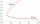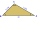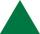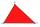# Length + inequalities - math problems

#### Number of problems found: 7

• Three-digit numbersHow many three-digit numbers are not closer to 600 on the number axis than to 400?
• The perimeterThe triangle has one side 5 cm long and the another 11 cm long. What can be the smallest and what is the largest perimeter?
• The perimeter of the rectangleThe length, l, of a rectangle is 4 inches greater than its width, w. The perimeter of the rectangle is at least 30 inches. What inequality shows the range of possible widths of the rectangle?
• TrianglesIvo wants to draw all the triangles whose two sides of which have a length of 4 cm and 9 cm, and the length of the third side is expressed in whole centimeters. How many triangles does he have?
• Built-up areaJohn build up area 5 x 7 = 35 m2 with building with a wall thickness 30 cm. How many centimeters would have to subtract from thickness of the walls that built-up area fell by 9%?
• QuizQAn isosceles triangle has two sides of length 7 km and 39 km. How long is a third side?
• TriangleFor how many integer values of x can 6, 19 and x be the lengths of the sides of triangle?

We apologize, but in this category are not a lot of examples.
Do you have an exciting math question or word problem that you can't solve? Ask a question or post a math problem, and we can try to solve it.

We will send a solution to your e-mail address. Solved examples are also published here. Please enter the e-mail correctly and check whether you don't have a full mailbox.

Do you want to convert length units? Length - math problems. Inequalities - math problems.